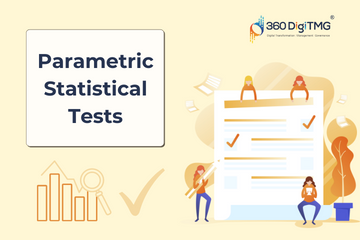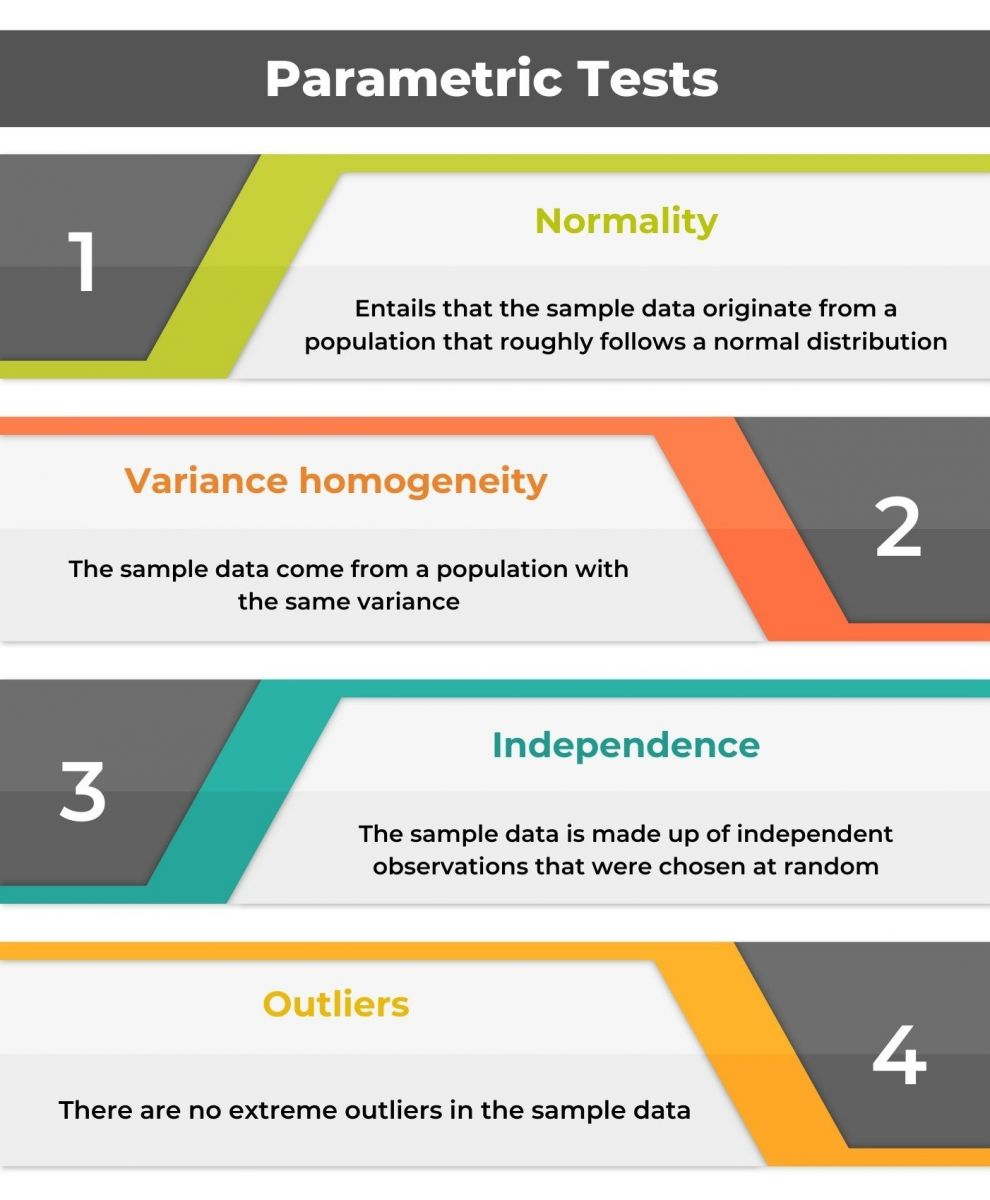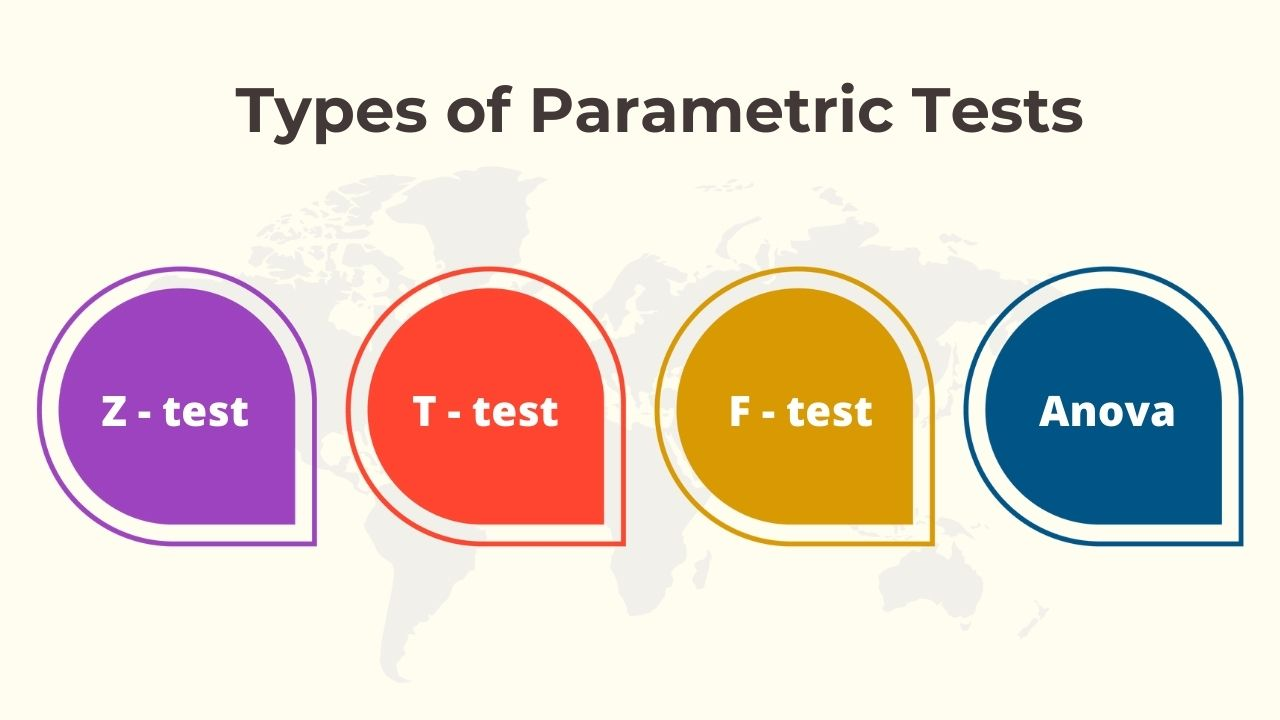Home / Blog / Data Science / Parametric Statistical Tests

# Parametric Statistical Tests

• June 28, 2023
• 5030
• 41### Meet the Author : Mr. Bharani Kumar

Bharani Kumar Depuru is a well known IT personality from Hyderabad. He is the Founder and Director of Innodatatics Pvt Ltd and 360DigiTMG. Bharani Kumar is an IIT and ISB alumni with more than 17 years of experience, he held prominent positions in the IT elites like HSBC, ITC Infotech, Infosys, and Deloitte. He is a prevalent IT consultant specializing in Industrial Revolution 4.0 implementation, Data Analytics practice setup, Artificial Intelligence, Big Data Analytics, Industrial IoT, Business Intelligence and Business Management. Bharani Kumar is also the chief trainer at 360DigiTMG with more than Ten years of experience and has been making the IT transition journey easy for his students. 360DigiTMG is at the forefront of delivering quality education, thereby bridging the gap between academia and industry.

The study of data is interdisciplinary. Data science is the analysis and generation of insights from data using statistics, mathematics, business intelligence, and computer programming that will be utilised as decision elements in strategic management. Jobs that statisticians once held are now being filled by data scientists. Data scientists need to be knowledgeable about statistical concepts, as well as how to apply key statistical equations, interpret, and communicate statistical results. The fundamental concepts of descriptive statistics, probability theory, over- and undersampling, dimensionality reduction, and Bayesian statistics must be understood by data scientists. Data science includes a variety of activities such as comprehending business difficulties, data scraping, data storage, data preparation, model construction, model deployment, and model monitoring. The many phases of data science activities need the use of mathematics and statistics. Understanding the statistical independence and form of the data distribution is aided by the testing of hypotheses. Hypothesis testing involves making assumptions and deciding whether or not to reject them. In machine learning, terminology like learning, hypothesis, instance, supervised learning, feature, and label are used in place of statistics terms like estimation, classifier, data point, regression, classification, covariate, and response.

### Parametric TestsDepending on the presumption that the population exhibits a normal distribution. There are parametric tests, such as simple linear regression, multilinear regression, and logistic regression, that use parameters like mean and variance to compare and assess disparity across sets of samples. Non-parametric tests are used to identify correlations between groups of data when metrics like mean and variance are not available.

### Learn the core concepts of Data Science Course video on Youtube:

As previously stated, parametric tests include a few assumptions that must be satisfied by the data:

• ### Normality -

entails that the sample data originate from a population that roughly follows a normal distribution.

• ### Variance homogeneity –

the sample data come from a population with the same variance.

• ### Independence –

the sample data is made up of independent observations that were chosen at random.

• ### Outliers –

there are no extreme outliers in the sample data.

• ### To solve the Business problem:

Data science initiatives begin by comprehending company issues and utilising statistics. whether or not the method used to resolve the business issue is important. Utilise a hypothesis test to demonstrate that the presumptions are producing meaningful outcomes.

• ### In Data Preprocessing:

Data preprocessing, which includes EDA, enables us to comprehend statistics of data, including minimum and maximum values, measures of central tendencies like mean, median, and mode, and measures of dispersion like variance, standard deviation, and range, as well as skewness and kurtosis.

Data preparation, which also incorporates statistics, is the process of preparing data for machine learning algorithms by managing outliers, normalising data, and transforming.

### Here are some statistical tests used in EDA

• To check for normality of data, we use the Shapiro test
• To find outliers, we use the Tietjen-Moore test
• To find the significance of the correlation, we use the t-test
• To find homogeneity of categorical variables, we use the chi-square test
• To find distribution, we use the Kolmogorov Smirnov test
• In Regression:
• To check heteroscedasticity, we use White’s test
• To check multicollinearity, we use the Farrar Glauber test
• To check if model coefficients are significant, we use values off-test
• To check the significance of the model, we use Anova /f-test for the decision

• ### Mean –

The measure of central tendency is the mean. The distribution's centre is shown by the mean.

The z-test and the t-test are the appropriate tests to apply when comparing the means of two groups.

How do we decide between a z-test and a t-test, though? by assessing the variation in the population and sample size.

A z-test is used when the sample size is large (greater than or equal to 30) and the population variance is known.

If the sample size is small (less than 30) and we are aware of the population variation, we can use either a z-test or a t-test.

When the population variance is unknown and the sample size is constrained, we utilise a t-test.

When the population variance is unknown and the sample size is large, we utilise a t-test.

• ### Variance -

Variance gives the distance between the mean and data points. It gives the dispersion of data.

If we wish to find equality between the two population variances we should go for a T-test.

The F-test is commonly used by researchers to determine whether or not two independent samples were selected from a normal population with the same variability.

If we wish to find equality of more than 2 population variances, we go for the ANOVA test. Click here to learn Data Science Course in Chennai

### Types of parametric tests• ### Z test:

A hypothesis test in which the z-statistic is normal is the z-test. The central limit theorem states that as the number of samples rises, it is expected that the samples are almost regularly distributed, hence the z-test works best for samples larger than 30.

The null and alternative hypotheses, alpha, and z-score should all be displayed when doing a z-test. The results and conclusion should be provided after computing the test statistic. A z-statistic, sometimes called a z-score, is a measurement of how many standard deviations a z-test result is above or below the population mean.

Z-tests include the one-sample location test, the two-sample location test, the paired difference test, and the maximum likelihood estimate.

• ### T-test:

The t-test is essentially similar to the z-test, with the exception that it performs better with smaller samples and does not need knowledge of population variation.

The t-test is based on the t-distribution, which is a bell-shaped curve with heavier tails than the normal distribution.

As the sample size grows, so do the degrees of freedom, and the t-distribution approaches the normal distribution. It gets less skewed and more compact around the mean (lighter tails).

T-tests are classified into three categories. one-sample and two-sample. Both of these fall under the umbrella of the 'unpaired t-test,' therefore the third form of the t-test is the 'paired t-test.'

Every statistical test has a test statistic that assists us in calculating the p-value, which decides whether or not to reject the null hypothesis. The test statistic in the instance of the t-test is known as the t-statistic. The formula for calculating the t-statistic varies based on the type of t-test used.

• ### F test

We may compare the variances of two sets of data using the F Test Formula. Calculate the mean and variance of the two provided data first before applying the F distribution under the null hypothesis.

F-testing to compare two variances, such as 1 and 2, a formula is used to divide them. The result will always be positive since the variances are always positive. As a result, the following F Test equation is used to compare two variances:

F = variation 1/variationtwo levels of freedom

Greater variance's DF (numerator) equals n1-1

Denominator: DF of lesser variance = n 2-1

The F value can be less than one in statistical calculations when the null hypothesis may be rejected, but it cannot be exactly equal to zero.

F cannot have its critical value equal to zero. If the F value is exactly 0, all samples have the same mean and same variance.

The fact that population variances are consistently considered to be equal is one of the most crucial things to understand when working with the F Statistic. The F value that was determined might not be accurate if this requirement is not met.

The results of the t-test and ANOVA are identical when there are just two samples. However, using a t-test when there are more than two samples would be inappropriate.

• ### Anova

Analysis of variance (ANOVA) is a statistical technique used to determine if the means of two or more groups differ substantially from one another. ANOVA compares the means of different samples to determine the influence of one or more factors.

ANOVA examines the means of various groups and determines whether or not there are statistical differences between the means. ANOVA is a type of omnibus test statistic. This means it cannot tell you which exact groups were statistically substantially different from one another, simply that at least two of them were.

It is critical to note that the primary ANOVA research issue is whether the sample means are from distinct populations. The ANOVA model is based on two assumptions:

First, regardless of the data collecting strategy used, the observations within each sampled population are normally distributed.

Second, the sampled population has a standard deviation of s2.

The one-way ANOVA examines the overall connection between the two variables, whereas the pairwise tests examine each potential pair of groups to check if one group has greater values than the other.

### Conclusion

Data scientists use a combination of computer algorithms and statistical equations to find patterns and trends in data. The relevance of such patterns and how they relate to actual situations are then examined utilising their knowledge of the social sciences and a particular industry or branch of business. The objective is to add value for a business or organisation.

### Data Analyst Courses in Other Locations